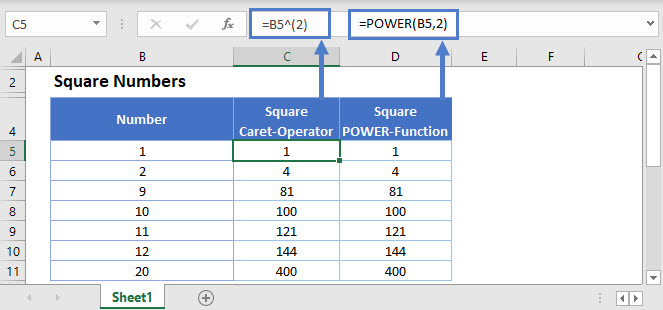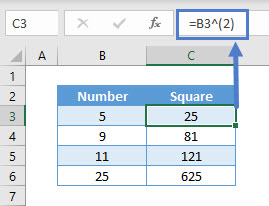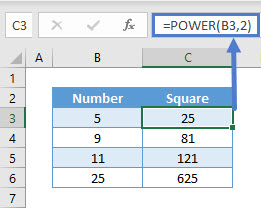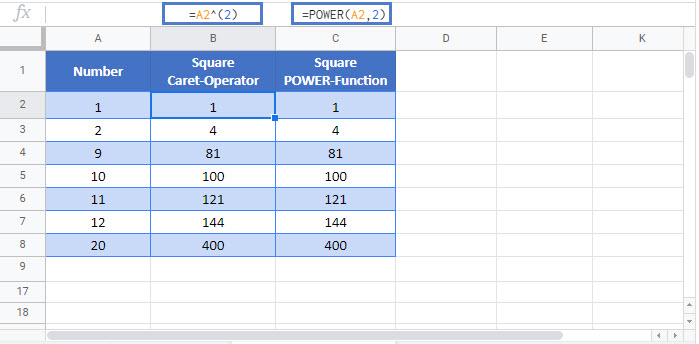# How to Square Numbers – Excel & Google Sheets

This tutorial will illustrate how to square a number in Excel & Google Sheets.## Square a Number using Caret Operator (^)

To square a number, you can either use the Caret/Exponent Operator (^) or the POWER function.

In this example, we use the Caret Operator.

``=B3^(2)``## Square a Number using POWER Function

The POWER function returns the result for a given number raised to a power. Its syntax is:

``=POWER(B3,2)``Negative numbers can be squared in a similar manner using both the functions.

Note: To take the square root of a number, simply use 1/2 instead of 2.

## Square Numbers in Google Sheets

These formulas work exactly the same in Google Sheets as in Excel### Excel Practice Worksheet

Practice Excel functions and formulas with our 100% free practice worksheets!